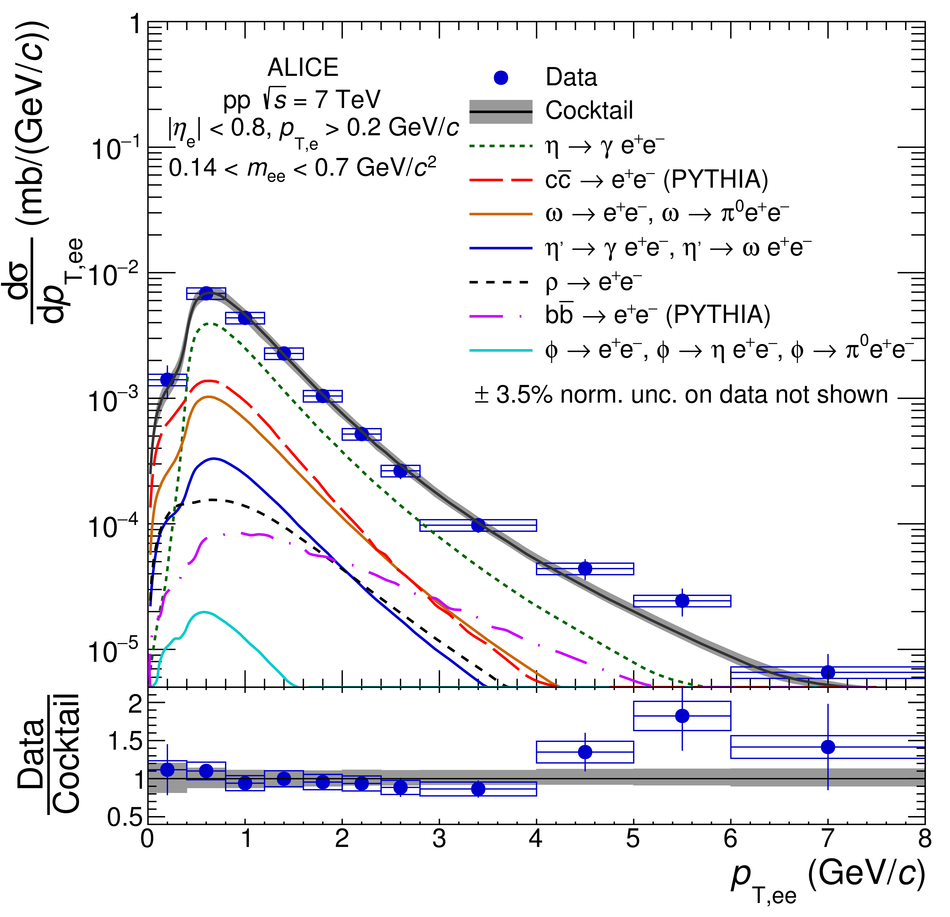Figure 11

 Inclusive \ee cross section in \pp collisions at \roots = 7\,TeV in the ALICE acceptance as a function of \ptee in the mass range 0.14 $<$ \mee $<$ 0.7~\GeVovercs (left) and 0.7 $<$ \mee $<$ 1.1~\GeVovercs (right). The data are compared with the hadronic cocktail. In the bottom panels, the ratios of data to cocktail as a function of \ptee are shown. Statistical and systematic uncertainties on the data are plotted as vertical bars and boxes, respectively. The total uncertainty of the cocktail is represented as a grey band.